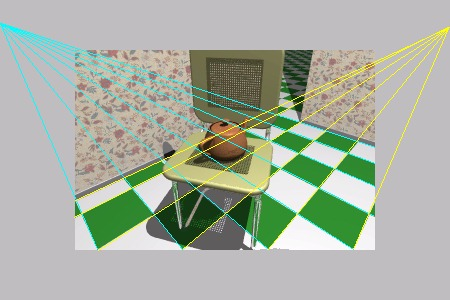# Perspective Correction

### Mappings that vary linearly in space do not vary linearly under perspective.#### The distance between parallel lines is an example. Another example is any mapping applied to the surfaces being rendered in projection (such as RGB color interpolation, or interpolation of the u,v coordinates of a texture map).

In order to correctly interpolate texture coordinates under perspective we must consider the set of all values that will be projected to be a single homogeneous vector:
(x, y, z, w, u, v, 1, R, G, B, A)
After transforming the vertex we have:
(xw, yw, zw, w, u, v, 1, R, G, B, A)
When projecting a homogenous vector we divide by the homogenous coordinate:
(xw/w, yw/w, zw/w, w/w, u/w, v/w, 1/w, R/w, G/w, B/w, A/w)
We then interpolate between the vertices and perform the projection of the texture coordinates at the pixels. That is, at the vertices we compute u/w, v/w, and 1/w. We linearly interpolate these three across the pixels. Then at each pixel we divide the interpolated texture coordinates by the interpolated 1/w to yield the final u,v.